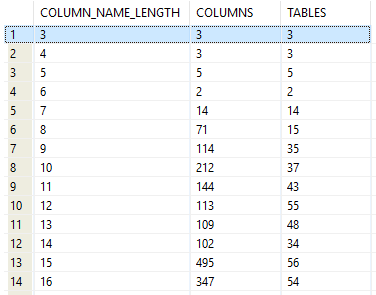## Get column name length distribution in Db2 database

Article for:

Query below returns distribution of column name lengths (number of characters).

## Query

``````select length(c.colname) as column_name_length,
count(*) as columns,
count(distinct c.tabschema concat '.' concat c.tabname) as tables
from syscat.columns c
inner join syscat.tables t on
t.tabschema = c.tabschema and t.tabname = c.tabname
where t.type = 'T'
and t.tabschema not like 'SYS%'
group by length(c.colname)
order by length(c.colname);
``````

## Columns

• column_name_length - lenght in characters of column name
• columns - number of columns with this length
• tables - number of tables that have columns with this name length

## Rows

• One row represents one name lenght (number of characters)
• Scope of rows: each column length that exists in a database
• Ordered by length ascending (from 1 to max)

## Sample results